# Rodrigues formula

(diff) ← Older revision | Latest revision (diff) | Newer revision → (diff)

A formula relating the differential of the normal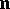to a surface to the differential of the radius vectorof the surface in the principal direction: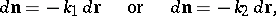whereandare the principal curvatures.

The formula was obtained by O. Rodrigues (1815).

A.B. Ivanov

A representation of orthogonal polynomials in terms of a weight function using differentiation. If a weight function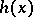satisfies a Pearson differential equation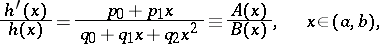and if, moreover, at the end points of the orthogonality interval the following conditions hold: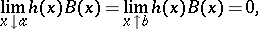then the orthogonal polynomialcan be represented by a Rodrigues formula:where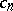is a constant. Rodrigues' formula holds only for orthogonal polynomials and for polynomials obtained from the latter by linear transformations of the argument. Originally, this formula was established by O. Rodrigues  for the Legendre polynomials.